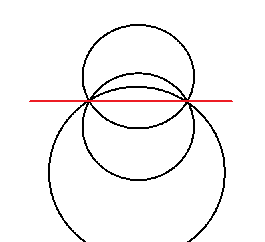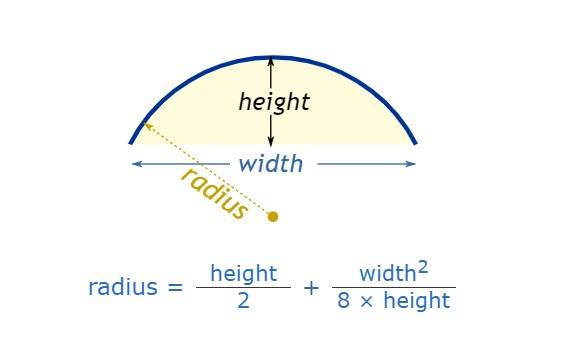# Do I have enough information to find the radius?

Godspanther
If there is a circle, 360 degrees, and 2 random points A & B are chosen on the circle. A straight line is drawn between those two points. You are not shown the whole circle. All you can see is A, B the straight line and an arc representing an unknown number of degrees. You know the length of the line from A to B. Assuming everything is to scale can one determine the radius of the circle or is more information needed?

No. If you construct the normal on the midpoint of the line, the center of the circle will be somewhere on that normal, but that is all.

Mentor
2022 Award
All you can see is A, B the straight line and an arc representing an unknown number of degrees.
The arc is the essential information here. What does it mean to know an arc of the circle? An arc has obviously at least three points which are enough to determine the circle.

•hutchphd, Lnewqban and pbuk
Homework Helper
Gold Member
You can resolve it graphically if you are able to precisely draw an isosceles triangle within the arc and cord area.

Godspanther
Damn. Now granted I suck at math, which really sucks since it is the language of reality itself, still I had hope. Since all circles are the same a person could draw a line at a 90 degree angle from the mid-point of line AB to the Crest of the arc. I had hope the there was a formula that one could use comparing the length of AB to the length of the midpoint line let's call that one C. If such a formula existed then one could draw a line of AB length in any size circle and be able to determine the circles size based on the length of line C. At least that is what I had hoped.

Homework Helper
Gold Member
Damn. Now granted I suck at math, which really sucks since it is the language of reality itself, still I had hope. Since all circles are the same a person could draw a line at a 90 degree angle from the mid-point of line AB to the Crest of the arc. I had hope the there was a formula that one could use comparing the length of AB to the length of the midpoint line let's call that one C. If such a formula existed then one could draw a line of AB length in any size circle and be able to determine the circles size based on the length of line C. At least that is what I had hoped.
That formula exists, it is used in construction.
Is this homework?

Mentor
2022 Award
The situation looks like this:If you have the arc length above the chord, then we are left with two possibilities. If we have a third point, then we have the circle.

Homework Helper
2022 Award
If one knows the sagitta then $$r=\frac { s^2+l^2} {2s}$$ where ##l=AB/2##

Last edited:
•Lnewqban
Godspanther
That formula exists, it is used in construction.
Is this homework?
Lol. No. I am 41 years old. I have a passing interest in forging. I am contemplating what would be the optimal degree of arc to create a perfect scimitar. The closest I can find online is this one. https://www.trueswords.com/Arabian-...vPlyZYonirFBQ-I4qoHK6Zjy3gk0mikuhmaxCVicPuhys But the blade is short. If I can find a formula I can print the picture, make the measurements and figure out the arc for a blade between 32-36 inches. I am still debating best blade length and will likely examine multiple designs.

•DaveE
Homework Helper
Gold Member
Lol. No. I am 41 years old. I have a passing interest in forging. I am contemplating what would be the optimal degree of arc to create a perfect scimitar. The closest I can find online is this one. https://www.trueswords.com/Arabian-...vPlyZYonirFBQ-I4qoHK6Zjy3gk0mikuhmaxCVicPuhys But the blade is short. If I can find a formula I can print the picture, make the measurements and figure out the arc for a blade between 32-36 inches. I am still debating best blade length and will likely examine multiple designs.
Very interesting project.
https://www.mathsisfun.com/geometry/arc.html•Banno, mathwonk and jim mcnamara
Godspanther
That is just the sort of thing I was looking for. That you very much.

•Lnewqban
Homework Helper
2022 Award
Known to all grinders of telescope mirrors as the Sagitta Theorem as I mentioned in #8 above. I bring it up because I have corrected my original post from ##~l=AB~##to ##~l=AB/2~## which comports with @Lnewqban result

First: I did not get the fact that you also have got the arc itself, not just the line AB. Second: The formula I quoted above is mathematically correct, but when the height is small relative to the width, the uncertainty in the radius calculation can easily be very large.

Homework Helper
2022 Award

uncertainty in the radius calculation can easily be very large.

Yes when grinding a telescope mirror you use it to calculate the depth of the sagitta required to produce a mirror of desired radius ( and therefore focus) from a circular glass blank. Commonly a "feeler" gauge of some sort is usually created to test "s" the sag depth as you grind (you call it "height"). Because you know l=AB/2 very accurately for the mirror blank the technique is quite precise.
But if you measure l with error ##\Delta l## then$$r=\frac { s^2+l^2} {2s}$$ $$\Delta r=\frac {\partial r } {\partial l} \Delta l$$ $$\Delta r=\frac l s \Delta l$$ As you have noted

Homework Helper
Gold Member
First: I did not get the fact that you also have got the arc itself, not just the line AB. Second: The formula I quoted above is mathematically correct, but when the height is small relative to the width, the uncertainty in the radius calculation can easily be very large.
You are absolutely correct; the method is as accurate as the measurements.
As you have seen, the OP just wants a rough estimate so he can shape a sword.
I have seen it used in buildings construction as well, where errors are not that critical either.

The formula for the uncertainty in r relative to the measurement error in l cited above is correct, but my problem lies with the measurement error in s. In that case, you have: $\Delta r=\frac{\partial r}{\partial s}\Delta s= \frac{4s^{2}-2(s^{2}+l^{2})}{4s^{2}}\Delta s = (\frac{1}{2}-\frac{l^{2}}{2s^{2}})\Delta s$ .

The formula for the uncertainty in r relative to the measurement error in l cited above is correct, but my problem lies with the measurement error in s. In that case, you have: $\Delta r=\frac{\partial r}{\partial s}\Delta s= \frac{4s^{2}-2(s^{2}+l^{2})}{4s^{2}}\Delta s = (\frac{1}{2}-\frac{l^{2}}{2s^{2}})\Delta s$ .
if the errors are independent $$(\Delta r)^2=|\frac{\partial r}{\partial s}|^2 (\Delta s)^2+|\frac{\partial r}{\partial l}|^2|\Delta l)^2$$
$$(\Delta r)^2=\left |1-\frac r s\right |^2~ (\Delta s)^2+\left|\frac l s\right |^2(~\Delta l)^2$$